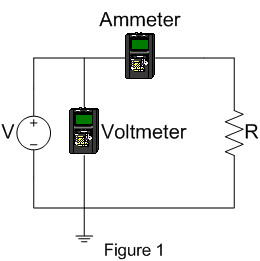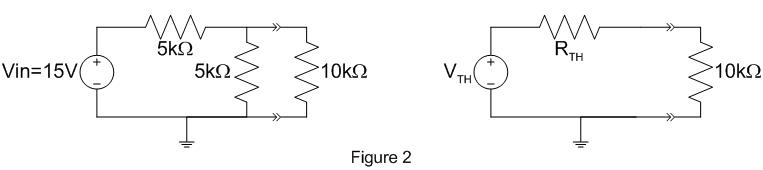EE 212 Lab

Lab 1: Resistors and the Multimeter

1. Current, I vs. Voltage, V for a Resistor.

Using a 22 kohm resistor, verify that Ohm's law is obeyed by measuring I and V for a few voltages. Use the adjustable 1.3-20 V dc supply and construct the circuit shown in figure 1 on your protoboard. Use two multimeters connected as shown to make the measurements. (Note that voltages are measured between points in the circuit, while currents are measured through a part of a circuit. Therefore you usually have to break the circuit to measure a current.) Be sure to use both positive and negative values of V. Does the display on the supply agree with your voltmeter? What does this say about measurement techniques in the lab? After you've taken at least ten measurments for the 22kohm resistor, try a 10kohm resistor. Plot the results for both resistors. Is Ohm's law obeyed for the resistors? From the plots, determine the values of the resistances. Compare to the values marked on the resistors, and to values measured with your multimeter.The voltmeter is not measuring the voltage at the place you want, namely across the resistor. Does that matter? How can you fix the circuit so the voltmeter measures what you want? Describe the impact of the voltmeter and ammeter internal resistances on the accuracy of the above measurements. For the instruments you are using, which connection is best?

2. I vs V for a Lamp.

Now perform the same measurements for a lamp keeping the voltage under 10V to protect the lamp from damage. Plot the results. What is the "resistance" of the lamp? What is the difference between the voltage-current relationship of the resistors and the lamp? Why are they different?

3. Thevenin Equivalent Circuit

Construct the voltage divider shown in the left portion of figure 2. Apply Vin = 15V and leave the 10 kohm load resistor off for now. Measure the (open circuit) output voltage across the load connection nodes. Next, measure the short circuit current. With this information, determine the Thevenin equivalent circuit. Attach the 10 kohm load resistor to the output. Measure the voltage across this load. Calculate the expected output voltage using the Thevenin equivalent circuit and compare it to the measured value.Keep the circuit you just built. Now build the Thevenin equivalent circuit shown in the right hand portion of figure 2 (without 10 kohm load resistor) using the variable  power supply and a resistor close to the required value. Check to see that its Thevenin voltage and Norton current are correct. Then attach the same 10kohm load resistor used above to see if the Thevenin equivalent circuit behaves the same as the original circuit.